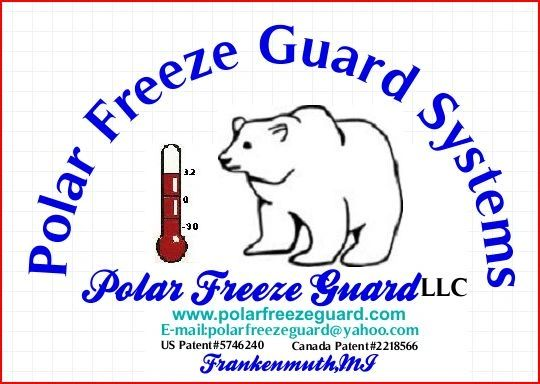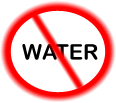Polar Freeze Guard LLCU.S. PATENT # 5746240

Weep Calculator

Weep Calculator

Below is a formula that you can use to see how much water and sewer that you are using from weep water.
Weep Calculator:

Water and Sewer Formula For Weep Water

Step 1:  # of bays x nozzle size(Gallons Per Minute) x 60 minutes = gallons per hour ( GPH)
Step 2:  # of hours of weep (below freezing) x GPH = Gallons of water & sewer used
Step 3:  GPH of water/sewer used divided by metered units (per 100, per 1000) = # of units
Step 4:  water & sewer rate x # of units = cost of water & sewer used to weep.

* Example only, cost savings will vary.

* Variables: nozzle size, water and sewer rates, water pressure, weep time & temperature

Example: 6-bay car wash, nozzle size 2505(.5 GPM), 9- days of weep

6 x .5 GPM = 3.0 GPM x 60 minutes = 180 GPH
216 hours x 180 GPH = 38,880 gallons
38,8880 Divided 1000 (units) = 38.88 units used
\$14.15 x 38.88 = \$550.15 ( cost of water & sewer used)

Use the calculator below to calculate your weep water used.